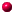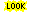´

# cycle index polynomial

The cycle index polynomial of given permutation group counts the number of group elements of a given cycle type. In Symmetrica there routines to do this computation for different finite groups. There are routines to compute the cycle index polynomial for the following permutation groups:symmetric groupalternating groupcyclic groupdihedral grouppermutation group given by generators